# Sample Data: Boston Homes

Housing values in suburbs of Boston

Originator: David A. Belsley, Edwin Kuh, and Roy E. Welsch

Home values for 506 Boston suburbs with potential influential factors (such as the crime rate, number of rooms, distance to employment centers, etc.). The test and training sets were obtained by creating a random sample of 30% of the data for the test set, using the rest for training.

## Examples

### Basic Examples

Retrieve the ResourceObject:

 In:=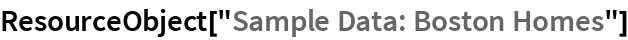Out=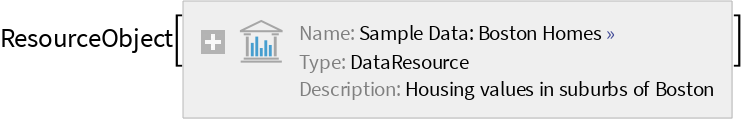View the data:

 In:=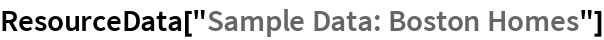Out=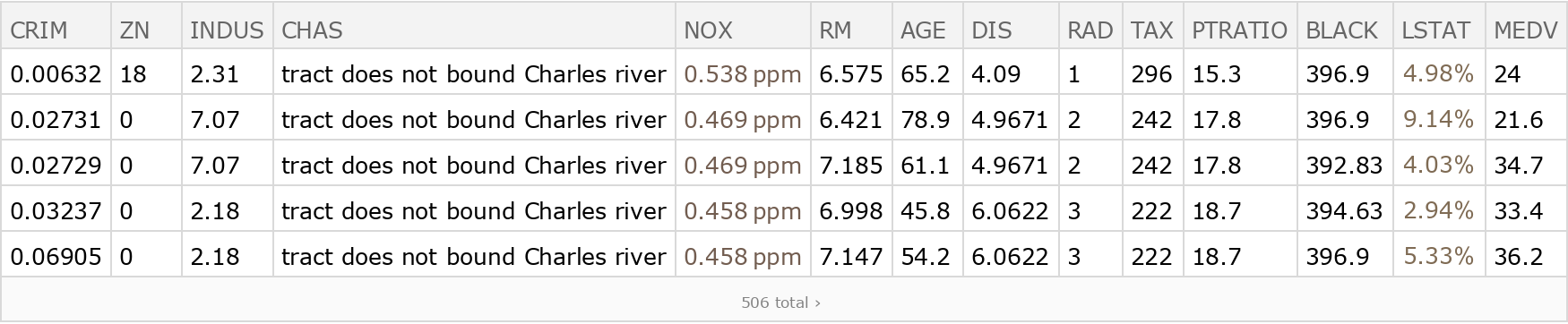### Visualization

Compare AGE to NOX values:

 In:=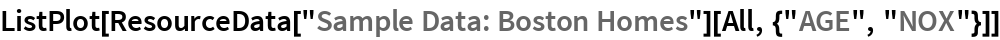Out=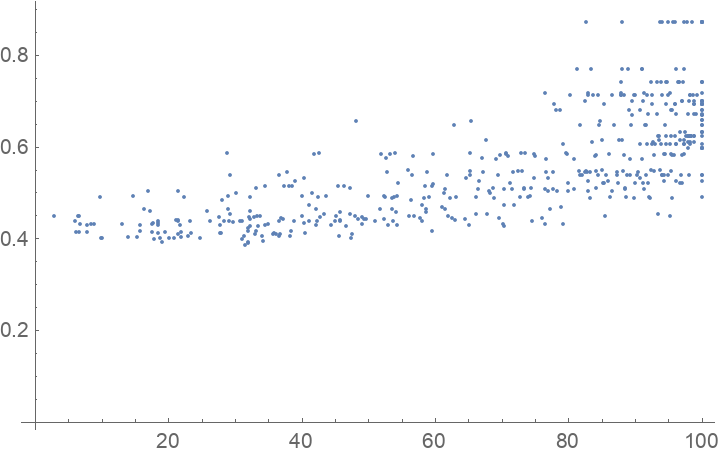### Analysis

Train a predictor:

 In:=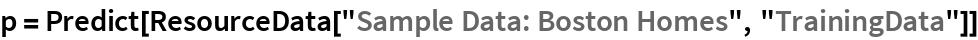Out=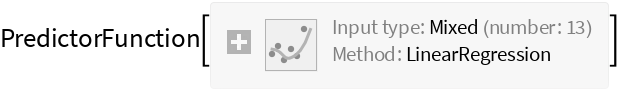Obtain general information about the predictor:

 In:=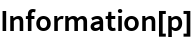Out=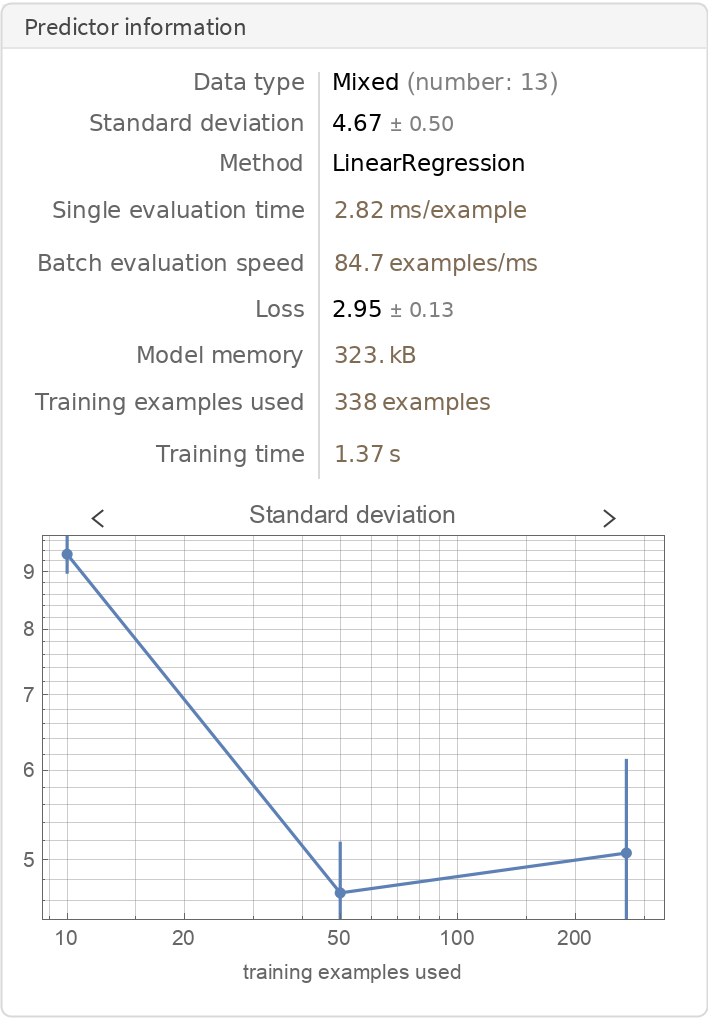Visualize the accuracy of the predictor on the test dataset:

 In:=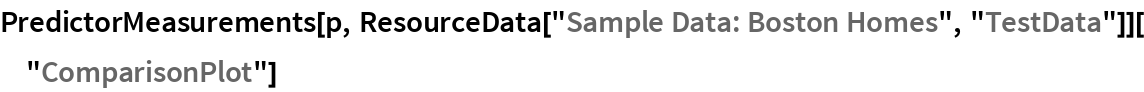Out=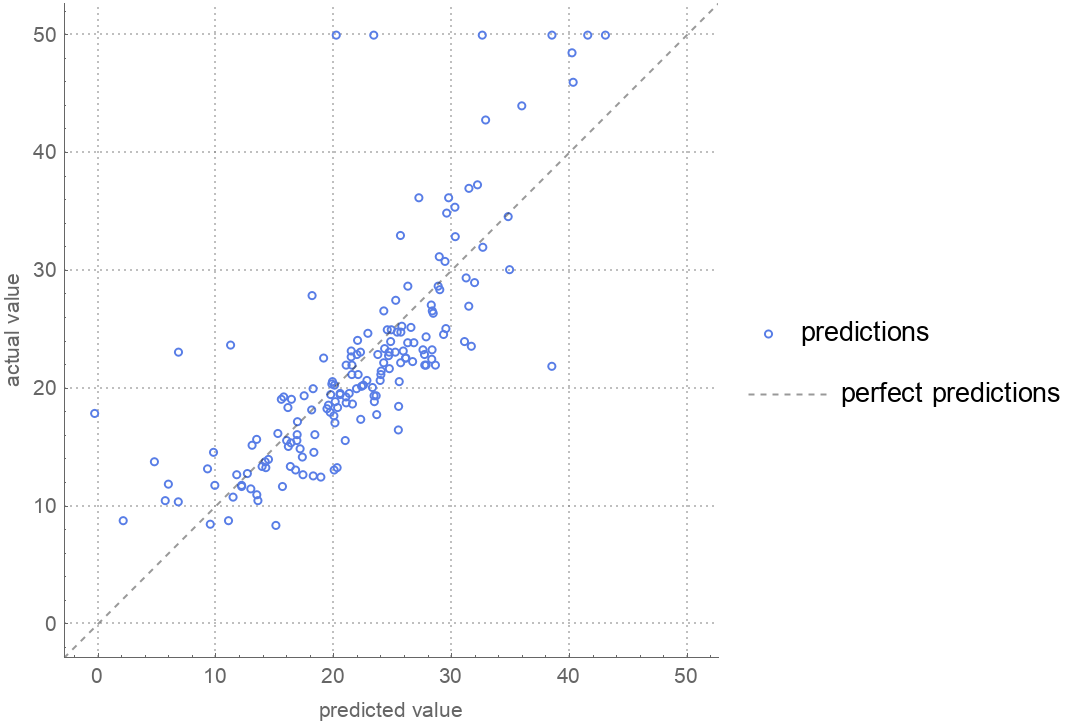Wolfram Research, "Sample Data: Boston Homes" from the Wolfram Data Repository (2019) https://doi.org/10.24097/wolfram.29771.data